# 个人博客转至：tybai.com# 【机器学习】算法原理详细推导与实现(七):决策树算法

## ID3决策树

### 信息熵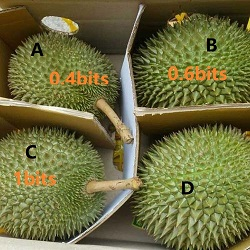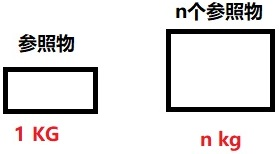$m=n\times x$

#### 均匀分布的信息熵

$n=\frac{m}{x}$

|正正正|反正正|
|正反正|正正反|
|反反正|反正反|
|正反反|反反反|


$n=log_xm$

$n=log_2m$

#### 一般分布的信息熵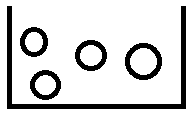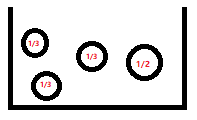$\frac{1}{6}log_2m_1+\frac{1}{6}log_2m_2+\frac{1}{6}log_2m_3+\frac{1}{2}log_2m_4$

$m_1=\frac{1}{\frac{1}{6}},m_2=\frac{1}{\frac{1}{6}},m_3=\frac{1}{\frac{1}{6}},m_4=\frac{1}{\frac{1}{2}}$

$\frac{1}{6}log_2\frac{1}{\frac{1}{6}}+\frac{1}{6}log_2\frac{1}{\frac{1}{6}}+\frac{1}{6}log_2\frac{1}{\frac{1}{6}}+\frac{1}{2}log_2\frac{1}{\frac{1}{2}}=1.79bit$

$H(X)=\sum_{i=1}^n p_ilog_2\frac{1}{p_i}$

### 信息增益

$H(X)=\sum_{i=1}^n p_ilog_2\frac{1}{p_i}$

$H(X,Y)=\sum_{i=1}^n p(x_i,y_i)log_2p(x_i,y_i)$

$\begin{split} H(X|Y)&=\sum_{y} p(y)H(X|Y=y) \\ &=-\sum_{y} p(y)\sum_{x}p(x|y)log(p(x|y)) \\ &=-\sum_{y}\sum_{x}p(x,y)log(p(x|y)) \\ &=-\sum_{x,y}p(x,y)log(p(x|y)) \end{split}$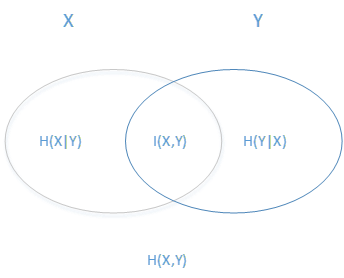$I(X,Y)=H(X)−H(X|Y)$

$\begin{split} H(X)&=\sum_{i=1}^n p_ilog_2\frac{1}{p_i} \\ &=\frac{1}{4}log_2\frac{1}{\frac{1}{4}}+\frac{1}{4}log_2\frac{1}{\frac{1}{4}}+\frac{1}{4}log_2\frac{1}{\frac{1}{4}}+\frac{1}{4}log_2\frac{1}{\frac{1}{4}} \\ &=2 \end{split}$

$\begin{split} H(X|Y)&=H(X1)+H(X2)+H(X3)+H(X4|Y=y) \\ &=\frac{1}{6}log_2\frac{1}{\frac{1}{6}}+\frac{1}{6}log_2\frac{1}{\frac{1}{6}}+\frac{1}{6}log_2\frac{1}{\frac{1}{6}}+\frac{1}{2}log_2\frac{1}{\frac{1}{2}} \\ &=1.79bit \end{split}$

$\begin{split} I(X,Y)&=H(X)−H(X|Y) \\ &=2-1.79 \\ &=0.21bit \end{split}$

### ID3决策树算法步骤

1. 计算$m$中的各个特征（一共$n$个）对输出$X$的信息增益，选择信息增益最大的特征$Y'$，其中$Y' \in Y$
2. 按特征$Y'$的不同取值$Y_i$将对应的样本输出$X$分成不同的类别$X_i$，其中$i \in n$。每个类别产生一个子节点。对应特征值为$Y_i$。返回增加了节点的数$T$
3. 对于所有的子节点，令$X=X_i,m=m−\{Y'\}$递归调用1-2步，得到子树$T_j$并返回。

## C4.5决策树

ID3算法虽然提出了新思路，但是还是有如下4点需要改进的地方：

1. ID3没有考虑连续特征，比如长度、密度都是连续值，无法在ID3运用，这大大限制了ID3的用途。--数据分箱即可解决
2. ID3采用信息增益大的特征优先建立决策树的节点，但信息增益准则对可取值数目较多的属性有所偏好。
3. 算法对于缺失值的情况没有做考虑。--特征工程即可解决
4. 没有考虑过拟合的问题。--预剪枝和后剪枝

$I(X,Y)=H(X)−H(X|Y)$

$\begin{split} H(X=外出|Y=大晴)&=\frac{1}{1}log_2\frac{1}{\frac{1}{1}}+0log_20 \\ &=0 \end{split}$

$Ir=(X,Y)=\frac{I(X,Y)}{IV(Y)}$

$IV(Y)=\sum_yp_ylogp_y$

$p_y$等于随机变量$Y=y$的概率。当信息熵$H(X|Y)$最小时，也就是分类各不相等的时候，$IV(Y)$也相对最小，这样就减轻了划分行为本身的影响。

## cart决策树

### 基尼系数$Gini=1-p_1^2-p_2^2-...-p_n^2$

$\{\frac{1}{10},\frac{2}{10},\frac{7}{10}\}$,$\{1,0,0\}$

$Gini=1-(\frac{1}{10})^2-(\frac{2}{10})^2-(\frac{7}{10})^2=0.46$

$Gini=1-(1)^2-(0)^2-(0)^2=0$

### 剪枝

• 预剪枝：在构造决策树的同时进行剪枝，也就是在节点划分前进行判断。所有决策树的构建方法，都是在无法进一步降低熵的情况下才会停止创建分支的过程，为了避免过拟合，可以设定一个阈值，熵减小的数量小于这个阈值，即使还可以继续降低熵，也停止继续创建分支。但是这种方法实际中的效果并不好。

• 后剪枝：在决策树生长完全构造好了过后，对树进行剪枝。剪枝的过程是对拥有同样父节点的一组节点进行检查，判断如果将其合并，熵的增加量是否小于某一阈值。如果确实小，则这一组节点可以合并一个节点。后剪枝是目前最普遍的做法。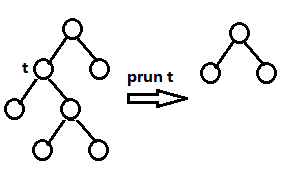#### Reduced-Error Pruning(REP,错误率降低剪枝）

REP是最简单粗暴的一种后剪枝方法，其目的减少误差样本数量。该算法是从下往上依次遍历所有的子树，直至没有任何子树可以替换使得在验证集上的表现得以改进时，算法就可以终止。

$f(T)=-\sum_{t \in T}e(t)$

$f(T')=-\sum_{t \in T'}e(t)$

$f(T) \leqslant f(T')$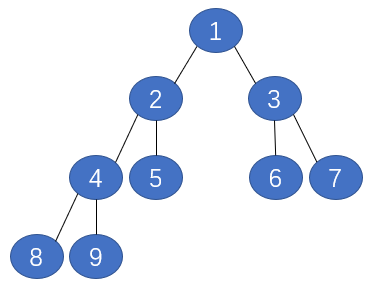Step 1： 将节点4删掉替换成8和9，测试在验证集上的表现，若表现更好，则将节点4删掉并替换成8和9的并集，若表现不好则保留原树的形状
Step 2： 将节点2删掉替换成8、9和5，测试在验证集上的表现
Step 3： 将节点3删掉替换成6和7，测试在验证集上的表现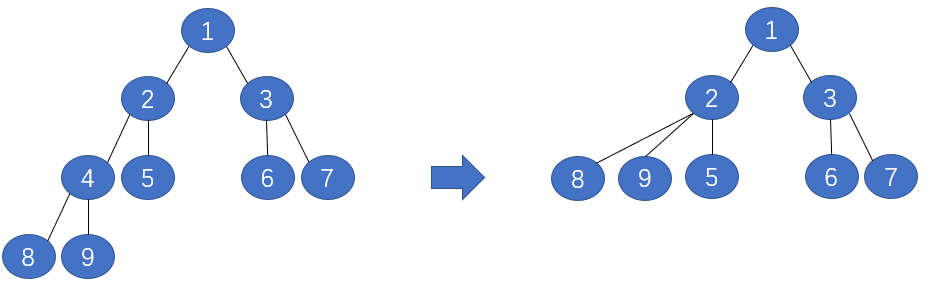REP是最简单的后剪枝方法之一，不过由于使用独立的验证集，如果数据量较少(数据要分为训练集、验证集、测试集三份)，那么在使用验证集进行剪枝的时候，可能会在验证集出现的稀有实例，却在测试集中没有出现，那么就会存在过度剪枝的情况。如果数据集较小，通常不考虑采用REP算法。尽管REP有这个缺点，但还是能够解决一定程度的过拟合问题。

#### Pesimistic-Error Pruning(PEP,悲观错误剪枝）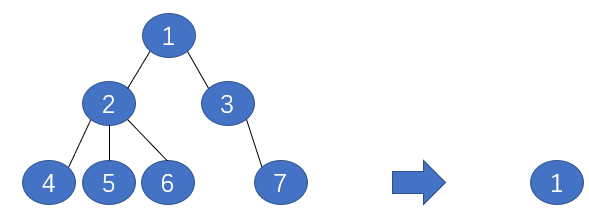$E(T) = \sum_{t \in T}\frac{e(t)+1/2}{N(t)}$

$E(T')=\sum_{t \in T, excep K}\frac{e(t)+1/2K}{N(t)}$

PEP悲观错误剪枝剪枝法定义，如果 E(剪枝后误判数均值)−E(剪枝前误判数均值)<std(剪枝前误判数标准差)，则可以剪枝，假设在子树中每一个样本的误判服从一个二项分布$B(N,p)$，其中$N$表示子树所包含的所有样本个数。

$e(t)=N(t)∗E(t)$

$std(t)=\sqrt{N(t)∗E(T)*(1-E(T))}$

$e(t')-e(t)<std(t)=\sqrt{N(t)\times E(T)\times (1-E(T))}$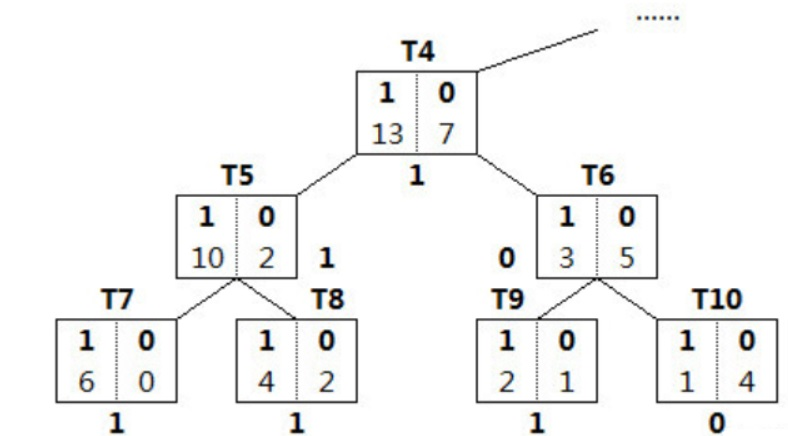$E(T)=\frac{(0+2+1+1)+4∗0.5}{20}=0.3$

$e(t)=20*0.3=6$

$std(t)=\sqrt{20*0.3*(1-0.3)}=2.05$

$E(T')=\frac{7+0.5}{20}=0.375$

$e(t')=20*0.375=7.5$

$7.5-2.05<6$

#### Cost-Complexity Pruning（CCP，代价复杂度剪枝)

$α= \frac{R(t)−R(T_t)}{∣N∣−1}​$

• $|N|$：子树$T_t$中的叶节点数;
• $R(t)$：结点$t$的错误代价，计算公式为$R(t)=r(t)*p(t)$;
• $r(t)$为结点t的错分样本率，$p(t)$为落入结点t的样本占所有样本的比例;
• $R(T_t)$：子树$T_t$错误代价，计算公式为$R(T_t)=\sum R(i)$$i$为子树$T_t$的叶节点。

CCP算法可以分为两个步骤，
Step 1： 按照上述公式从下到上计算每一个非叶节点的αα值，然后每一次都剪掉具有最小αα值的子树。从而得到一个集合$\{T_0,T_1,T_2,...,T_M\}$，其中，$T_0$表示完整的决策树，$T_M$表示根节点
Step 2： 根据真实的错误率在集合$\{T_0,T_1,T_2,...,T_M\}$选出一个最好的决策树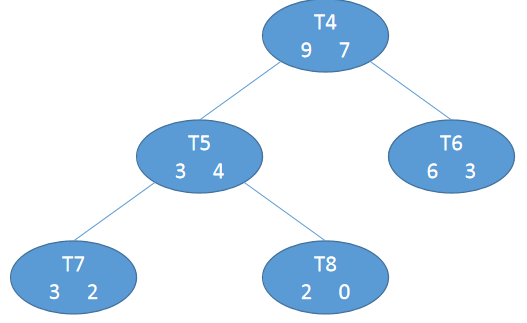$R(t)=r(t)*p(t)=\frac{7}{9+7}*\frac{9+7}{100}=\frac{7}{100}$

$R(T_t)=\sum R(i)=R(7)+R(8)+R(6)=\frac{2}{3+2}*\frac{3+2}{100}+\frac{0}{0+2}*\frac{0+2}{100}+\frac{3}{6+3}*\frac{6+3}{100}=\frac{5}{100}$

$α= \frac{R(t)−R(T_t)}{∣N∣−1}​=\frac{\frac{7}{100}-\frac{5}{100}}{3-1}=\frac{1}{100}$

REP PEP CCP

## 总结

ID3 分类 信息增益 不支持 不支持 不支持
C4.5 分类 信息增益比 支持 支持 支持
CART 分类、回归 基尼系数 支持 支持 支持

## 实例

### 计算逻辑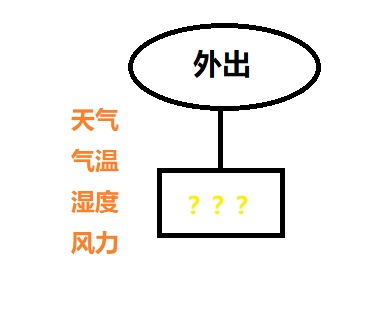$\sum p_ilog_2\frac{1}{p_i}$

$\begin{split} H(X=外出)&=\frac{9}{14}log_2\frac{1}{\frac{9}{14}}+\frac{5}{14}log_2\frac{1}{\frac{5}{14}} \\ &=0.940 \end{split}$

• 天气为晴朗时，2/5的概率外出，3/5的概率不外出，晴朗的信息熵$H(X=外出|Y=晴朗)$为:

$\begin{split} H(X=外出|Y=晴朗)&=\frac{2}{5}log_2\frac{1}{\frac{2}{5}}+\frac{3}{5}log_2\frac{1}{\frac{3}{5}} \\ &=0.971 \end{split}$

• 天气为多云时，0的概率外出，0的概率不外出，信息熵$H(X=外出|Y=多云)$为:

$\begin{split} H(X=外出|Y=多云)&=0+0 \\ &=0 \end{split}$

• 天气为下雨时，3/5的概率外出，2/5的概率不外出，信息熵$H(X=外出|Y=下雨)$为:

$\begin{split} H(X=外出|Y=下雨)&=\frac{3}{5}log_2\frac{1}{\frac{3}{5}}+\frac{2}{5}log_2\frac{1}{\frac{2}{5}} \\ &=0.971 \end{split}$

$\begin{split} H(X=外出|Y=天气)&=\frac{5}{14} \times H(X=外出|Y=晴朗)+0 \times H(X=外出|Y=多云)+\frac{4}{14} \times H(X=外出|Y=下雨) \\ &=\frac{5}{14} \times 0.971+0 \times 0+\frac{4}{14} \times 0.971 \\ &=0.693 \end{split}$

天气的信息增益$I(X=外出,Y=天气)$为：

$\begin{split} I(X=外出,Y=天气)&=H(X=外出)-H(X=外出|Y=天气) \\ &=0.940-0.693 \\ &=0.247 \end{split}$

$\begin{split} H(X=外出|Y=气温)&=\frac{4}{14}H(X=外出|Y=高温)+\frac{6}{14}H(X=外出|Y=温暖)+\frac{4}{14}H(X=外出|Y=寒冷) \\ &=\frac{4}{14}(\frac{2}{4}log_2\frac{1}{\frac{2}{4}}+\frac{2}{4}log_2\frac{1}{\frac{2}{4}})+\frac{6}{14}(\frac{4}{6}log_2\frac{1}{\frac{4}{6}}+\frac{2}{6}log_2\frac{1}{\frac{2}{6}})+\frac{4}{14}(\frac{3}{4}log_2\frac{1}{\frac{3}{4}}+\frac{1}{4}log_2\frac{1}{\frac{1}{4}}) \\ &=\frac{4}{14}\times 1.0+\frac{6}{14}\times 0.918296+\frac{4}{14}\times 0.811278 \\ &=0.911 \end{split}$

气温的信息增益$I(X=外出,Y=气温)$为：

$\begin{split} I(X=外出,Y=气温)&=H(X=外出)-H(X=外出|Y=气温) \\ &=0.940-0.911 \\ &=0.029 \end{split}$

湿度的信息熵$H(X=外出|Y=湿度)$为：

$\begin{split} H(X=外出|Y=湿度)&=\frac{7}{14}H(X=外出|Y=高)+\frac{7}{14}H(X=外出|Y=正常) \\ &=\frac{7}{14}\times (\frac{6}{7}log_2\frac{1}{\frac{6}{7}}+\frac{1}{7}log_2\frac{1}{\frac{1}{7}})+\frac{7}{14}\times (\frac{3}{7}log_2\frac{1}{\frac{3}{7}}+\frac{4}{7}log_2\frac{1}{\frac{4}{7}}) \\ &=\frac{7}{14}\times 0.591673+\frac{7}{14}\times 1.159856 \\ &=0.788 \end{split}$

湿度的信息增益$I(X=外出,Y=湿度)$为：

$\begin{split} I(X=外出,Y=湿度)&=H(X=外出)-H(X=外出|Y=湿度) \\ &=0.940-0.788 \\ &=0.152 \end{split}$

风力的信息熵$H(X=外出|Y=风力)$为：

$\begin{split} H(X=外出|Y=风力)&=\frac{6}{14}E_{有风}+\frac{8}{14}E_{无风} \\ &=\frac{6}{14}\times (\frac{3}{6}log_2\frac{1}{\frac{3}{6}}+\frac{3}{6}log_2\frac{1}{\frac{3}{6}})+\frac{8}{14}\times (\frac{6}{8}log_2\frac{1}{\frac{6}{8}}+\frac{2}{8}log_2\frac{1}{\frac{2}{8}}) \\ &=\frac{6}{14}\times 1.0+\frac{8}{14}\times 0.811278 \\ &=0.892 \end{split}$

风力的信息增益$I(X=外出,Y=风力)$为：

$\begin{split} I(X=外出,Y=风力)&=H(X=外出)-H(X=外出|Y=风力) \\ &=0.940-0.892 \\ &=0.048 \end{split}$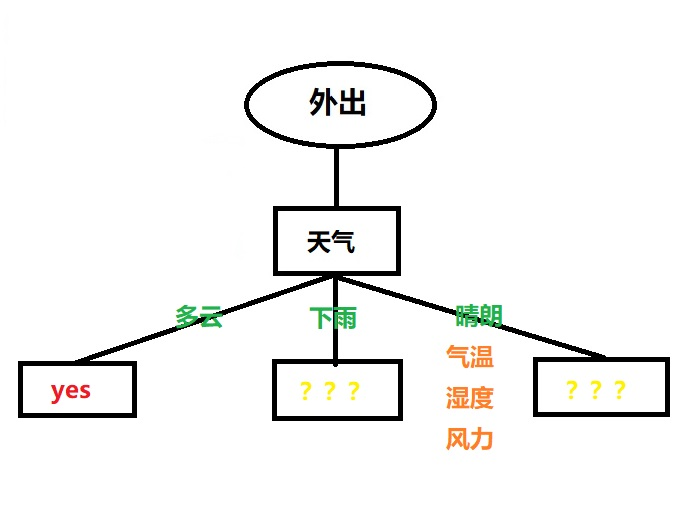天气=下雨的情况下：

气温的信息熵$H(X_1=外出,X_2=下雨|Y=气温)$为：

$\begin{split} H(X_1=外出,X_2=下雨|Y=气温)&=H(X_1=外出,X_2=下雨|Y=温暖)+H(X_1=外出,X_2=下雨|Y=寒冷) \\ &=\frac{3}{5}\times (\frac{2}{3}log_2\frac{1}{\frac{2}{3}}+\frac{1}{3}log_2\frac{1}{\frac{1}{3}})+\frac{2}{5}\times (\frac{1}{2}log_2\frac{1}{\frac{1}{2}}+\frac{1}{2}log_2\frac{1}{\frac{1}{2}}) \\ &=0.951 \end{split}$

天气=晴朗的情况下：

$H(X_1=外出,X_2=晴朗|Y=湿度)$最大，那么新得到的树形如下所示：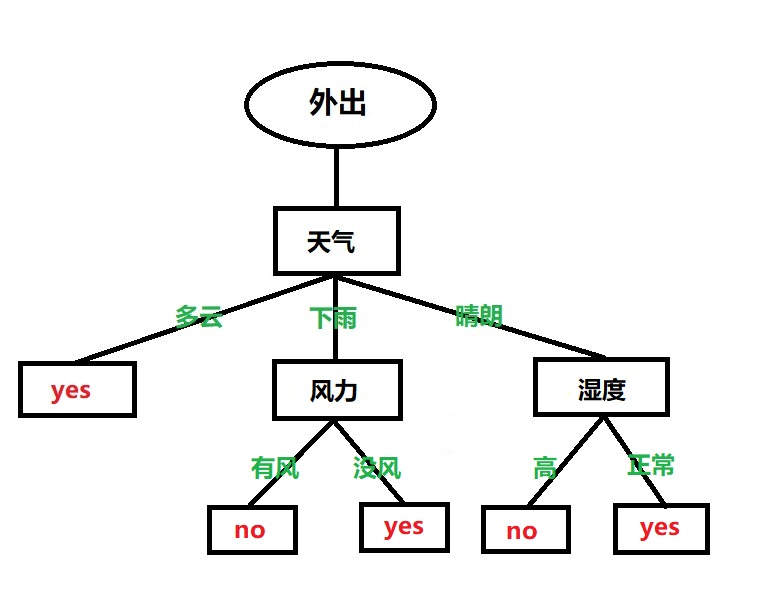### 计算代码

data[data == u'晴朗'] = 2
data[data == u'多云'] = 1
data[data == u'下雨'] = 0
data[data == u'高温'] = 2
data[data == u'温暖'] = 1
data[data == u'寒冷'] = 0
data[data == u'高'] = 1
data[data == u'正常'] = 0
data[data == u'有风'] = 1
data[data == u'无风'] = 0
data[data == u'是'] = 1
data[data == u'否'] = 0


# 建立决策树模型，基于信息熵
dtc = DTC(criterion='entropy')
dtc.fit(x_train, y_train)


print(dtc.predict(x_test))
print(dtc.score(x_test, y_test))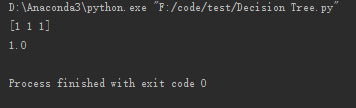posted on 2020-08-22 08:23  TTyb  阅读(296)  评论(0编辑  收藏

### 导航